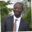Related Tags

javascript
algorithm
communitycreator

# How to sum up all the numbers in a range in JavaScriptAbel Lifaefi Mbula

### Overview

In this shot, we’ll discuss three ways to sum up all the numbers in a given range in JavaScript.

Our program should accept an array of two numbers as input, for example, sumAll([1, 3]). Then, it should process the sum of all the numbers in the given range. It should be noted that both the numbers in the given range are inclusive, that is, 1 and 3 in this example. Finally, the program should return the total/sum as output, which is 6 in the case of this example.

The lowest number will not always come first. Hence, [3,1] should be treated as [1,3].

The workflow of our program

### How to use the traditional for loop

function sumAll(arr) {
if (arr > arr) // if the highest number is on the left, sort the array
arr.sort((a, b) => a - b)

let sum = 0 // Initialize a variable that will be holding the sum

for (let i = arr; i <= arr; i++) // Loop over the range
sum += i

return sum // return result
}

console.log(sumAll([3,1]))
Testing the "for" loop implementation

### How to use the .reduce() method

Here we will implement:

function sumAll(arr) {
if (arr > arr)
arr.sort((a, b) => a - b)

const newArr = []

for(let i = arr; i <= arr; i += 1)
newArr.push(i)

return newArr.reduce((acc, cur) => acc + cur)
}

console.log(sumAll([3,1]))
Testing the "reduce()" implementation

We can also use methods other than reduce() to get the sum of the newly created array items.

Happy coding!

RELATED TAGS

javascript
algorithm
communitycreator

CONTRIBUTORAbel Lifaefi Mbula
RELATED COURSES

View all Courses

Keep Exploring

Learn in-demand tech skills in half the time